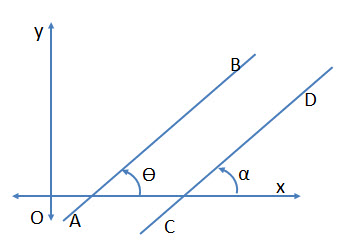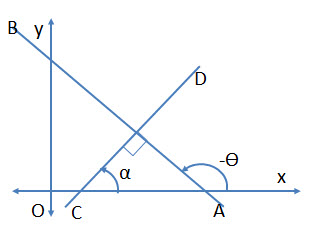Basic Concept of a Line

• Any point, that satisfies the equation of a line, will lie on the line.
• Also, any point through which a line passes, will always satisfy the equation of that line.
• Every straight line can be represented by a linear equation in two dimensions ($x \ and \ y$ plane).

Inclination of a Line

The angle$\theta$ that the line makes with the$x-axis$ is called the “inclination of the line”. The angle$\theta$ is when measured in anti-clockwise direction the inclination is  positive and if measured clock-wise then the inclination is negative.

Note:

• Inclination angle$\theta$ for$x-axis$ is$0^o$ . Also all lines parallel to$x-axis$ will also have the inclination angle as$0^o$ .
• Inclination angle$\theta$ for$y-axis$ is$90^o$ . Also all lines parallel to$y-axis$ will also have the inclination angle as$90^o$ .

Slope (or Gradient) of a Line

The slope of the line is the tangent of its inclination and is denoted by$m$ i.e. Slope$m = \tan \theta$

Therefore

Slope  of the$x-axis = \tan 0^o= 0$

Slope of the$y-axis = \tan 90^o = \infty$ (not defined)The slope of the line is positive if the line makes an acute angle with$x-axis$ when measured in anti-clock wise direction  i.e.$\tan \theta$ is positive.

The slope of the line is negative if the line makes and obtuse angle with$x-axis$ when measured in anti-clockwise direction. i.e.$\tan \theta$ is negative.

Formula for “Slope of Line passing through two points”

Let the two points be$(x_1, y_1)$ and$(x_2, y_2)$$\displaystyle m = \tan \theta = \frac{y_2-y_1}{x_2-x_1} = \frac{y_1-y_2}{x_1-x_2}$

Parallel LinesTwo lines having inclination as$\theta \ and \ \alpha$  will be parallel if$\alpha=\theta \ or \ \tan \alpha=tan\theta$

i.e. slope of the two lines is equal and hence they are parallel lines.

Perpendicular LinesTwo lines having inclination as$\theta$ and$\alpha$ will be perpendicular if:$\theta = 90^o+\alpha$$\Rightarrow \tan \theta=tan \ (90^o+\alpha)$$\Rightarrow \tan \theta=- \cot \alpha$$\displaystyle \Rightarrow \tan \theta=- \frac{1}{\tan \alpha}$$\Rightarrow \tan \theta \times \tan \alpha = -1$

or$m_1 \times m_2 = -1$ where$m_1 \ and \ m_2$ are slopes of the two lines respectively.

Note:

• If two lines are perpendicular, then the product of their slopes is$-1$. Conversely, if the product of the two lines is$-1$, they are perpendicular.
• Example: If the slope of a line is$2$, then the slope of another line parallel to this line would be$2$. Also the slope of a line perpendicular to this line would be$- \frac{1}{2}$.
• A line whose slope is$m=0$, is parallel to$x-axis$.
• Also the slope of$y-axis$  is not defined, all likes parallel to$y-axis$ will have slope which is not defined.

Condition for Collinearity of Three Points

Let there be three points$A(x_1, y_1), B(x_2, y_2) \ and \ C(x_3, y_3)$

If the slope of segment AB and BC is the same, then they are all on the same line and are collinear.$\displaystyle m = \frac{y_2-y_1}{x_2-x_1} = \frac{y_3-y_2}{x_3-x_2}$

X-intercepts & Y-intercepts

Refer to first figure.

If a line meets the$x-axis$, then the distance from origin to the point of intercept is called$x-intercept$.

Similarly, if a line meets the$y-axis$, then the distance from origin to the point of intercept is called$y-intercept$.

Equation of a Line

Slope-Intercept Form: When the slope$(m)$ is given and the intercept on the$y-axis$ is given.

The the equation is:$y = mx+c$ where$m$ is the slope and$c = intercept \ on \ y-axis$.

Point-Slope form: When the slope of the line and a point$(x_1, y_1)$ on a line is given

The equation is:$y-y_1 = m(x-x_1)$

Equally Inclined Lines

This means that the lines make equal angle from both the coordinate axes. As shown in the figure, lines$AB \ and \ CD$ are equally inclined.

For$AB: Inclination \ \theta=45^o$. Therefore slope$= \tan 45^o = 1$

For$CD: Inclination \ \theta=-45^o$. Therefore the slope$= \tan (-45^o) = -1$